# Math > Year 2

## Combining amounts and coins

Let’s learn about the pounds and pence.

Pounds and Pence are used for measuring the money.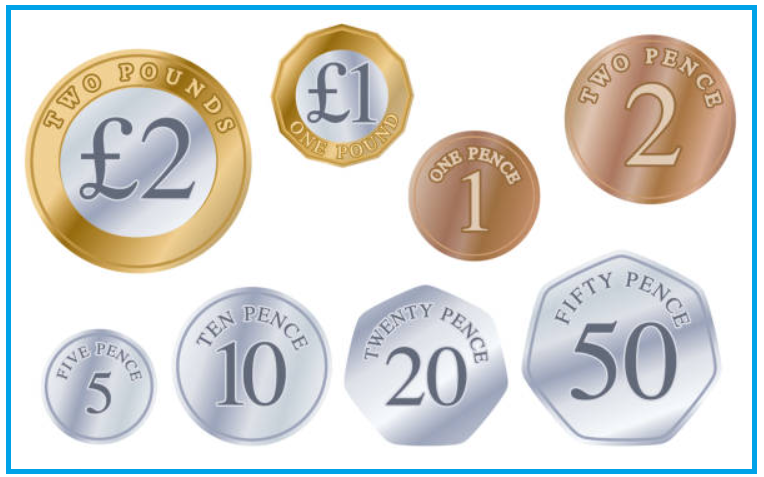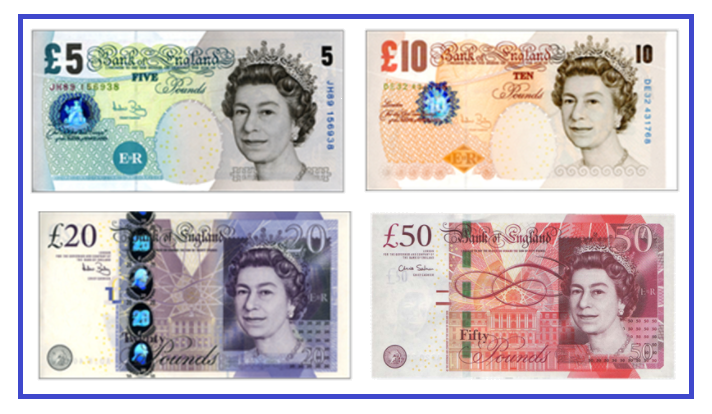Pounds are in the form of notes and coins.

Pence are only in the form of coins

Symbols to represent pounds and pence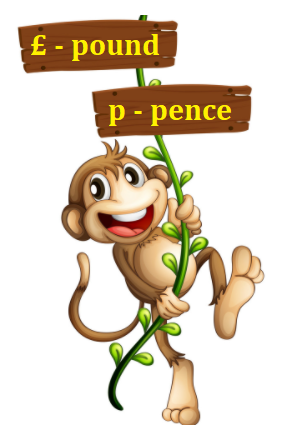Combination of amounts and coins:

£1 is the same as 100 p.

Some combination of amounts to make £1:

2 coins of 50 pence are equal to 1 pound.

2 coins of 50p = 100p

100 p = £1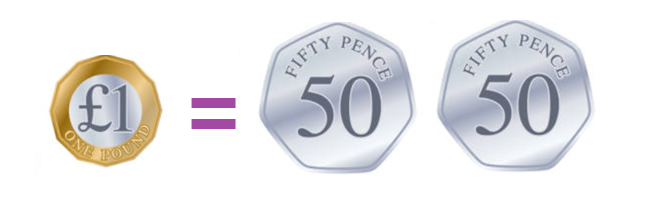5 coins of 20 pence are equal to 1 pound.

5 coins of 20p = £1

20 p + 20p + 20p + 20p + 20p = £1

100 p =£1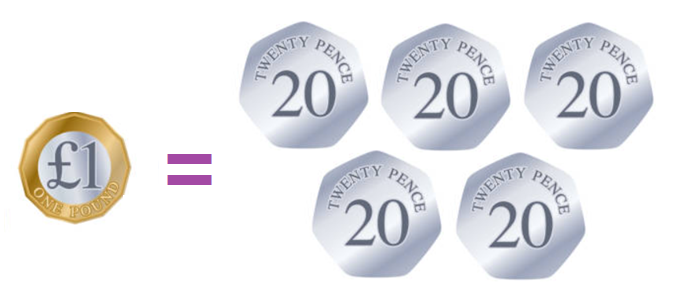10 coins of 10 pence are equal to 1 pound.

10 coins of 10p = £1

10 p + 10p + 10p + 10p + 10p + 10 p + 10p + 10p + 10p + 10p = £1

100 p = £1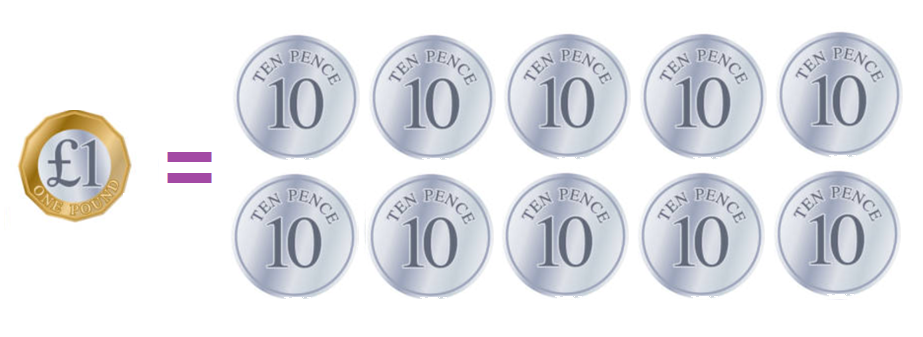Some combination of amounts to make £2:

4 coins of 50 pence are equal to 1 pound.

4 coins of 50p = £2

50 p + 50p + 50p + 50p = £2

200 p = £2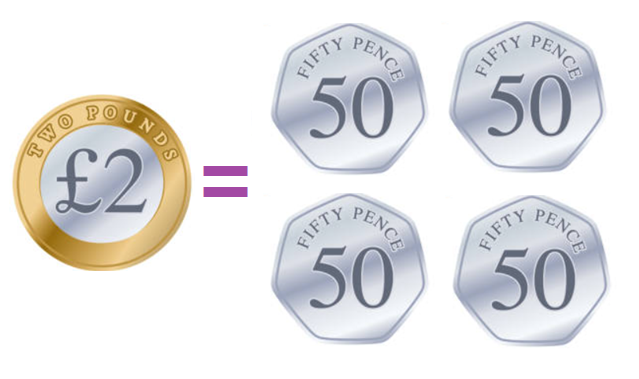10 coins of 20 pence are equal to 2 pounds.

10 coins of 20p = £2

20 p + 20p + 20p + 20p + 20p + 20p + 20p + 20p + 20p + 20p = £2

200 p = £2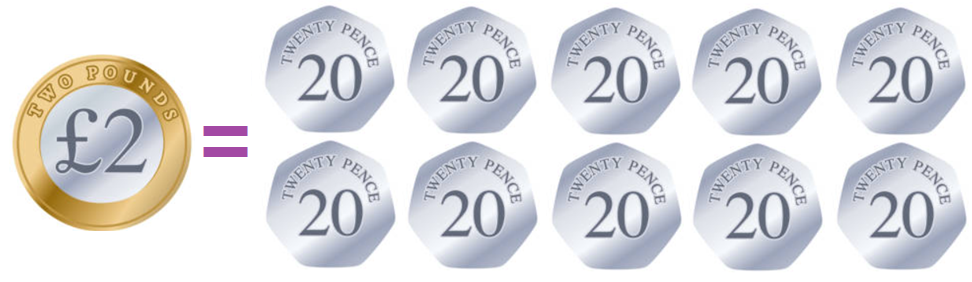10 coins of 20 pence are equal to 2 pounds.

20 coins of 10p = £2

10 p + 10p + 10p + 10p + 10p + 10p + 10p + 10p + 10p + 10p + 10 p + 10p + 10p + 10p + 10p + 10p + 10p + 10p + 10p + 10p = £2

200 p = £2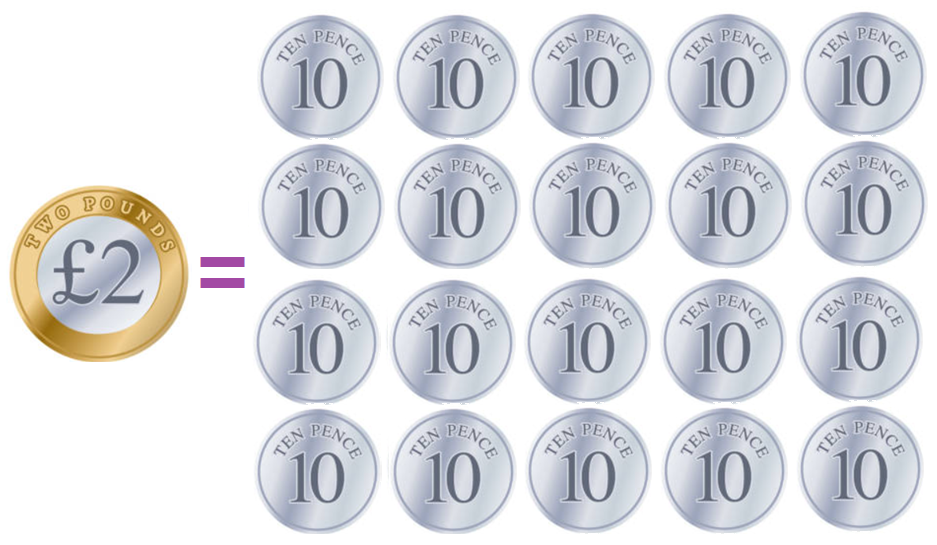Let’s try a question:

Question: How many coins of 10p are equal to £1?

Explanation:

10 coins of 10p = £1

10 p + 10p + 10p + 10p + 10p + 10 p + 10p + 10p + 10p + 10p = £1

100 p = £1

//